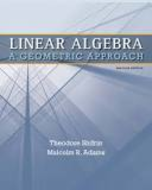### Solution Found!# Give Cartesian equations of the given hyperplanes: a. x = (1, 2) + t (3, 2) b. The plane

Chapter 1, Problem 1

(choose chapter or problem)

Get Unlimited Answers! Check out our subscriptions
QUESTION:

Give Cartesian equations of the given hyperplanes:

a. $$\text { a. } \mathbf{x}=(-1,2)+t(3,2)$$

b. The plane passing through (1, 2, 2) and orthogonal to the line $$\mathbf{x}=(5,1,-1)+t(-1,1,-1)$$

c. The plane passing through (2, 0, 1) and orthogonal to the line $$\mathbf{x}=(2,-1,3)+t(1,2,-2)$$

d. The plane spanned by (1, 1, 1) and (2, 1, 0) and passing through (1, 1, 2)

e. The plane spanned by (1, 0, 1) and (1, 2, 2) and passing through $$(-1,1,1)$$

f. The hyperplane in $$\mathbb{R}^{4}$$ through the origin spanned by $$(1,-1,1,-1),(1,1,-1,-1)$$, and $$(1,-1,-1,1)$$.

### Questions & Answers (3 Reviews)

QUESTION:

Give Cartesian equations of the given hyperplanes:

a. $$\text { a. } \mathbf{x}=(-1,2)+t(3,2)$$

b. The plane passing through (1, 2, 2) and orthogonal to the line $$\mathbf{x}=(5,1,-1)+t(-1,1,-1)$$

c. The plane passing through (2, 0, 1) and orthogonal to the line $$\mathbf{x}=(2,-1,3)+t(1,2,-2)$$

d. The plane spanned by (1, 1, 1) and (2, 1, 0) and passing through (1, 1, 2)

e. The plane spanned by (1, 0, 1) and (1, 2, 2) and passing through $$(-1,1,1)$$

f. The hyperplane in $$\mathbb{R}^{4}$$ through the origin spanned by $$(1,-1,1,-1),(1,1,-1,-1)$$, and $$(1,-1,-1,1)$$.

Step 1 of 7

We will get the Cartesian equations of each of the given hyperplanes above.

### Review this written solution for 964011) viewed: 507 isbn: 9781429215213 | Linear Algebra: A Geometric Approach - 2 Edition - Chapter 1.3 - Problem 1

Thank you for your recent purchase on StudySoup. We invite you to provide a review below, and help us create a better product.

Textbook: Linear Algebra: A Geometric Approach

Click to rate

Write a review below (optional):

### Review this written solution for 964011) viewed: 507 isbn: 9781429215213 | Linear Algebra: A Geometric Approach - 2 Edition - Chapter 1.3 - Problem 1

Thank you for your recent purchase on StudySoup. We invite you to provide a review below, and help us create a better product.

Textbook: Linear Algebra: A Geometric Approach

Click to rate

Write a review below (optional):

### Review this written solution for 964011) viewed: 507 isbn: 9781429215213 | Linear Algebra: A Geometric Approach - 2 Edition - Chapter 1.3 - Problem 1

Thank you for your recent purchase on StudySoup. We invite you to provide a review below, and help us create a better product.

Textbook: Linear Algebra: A Geometric Approach

Click to rate

Write a review below (optional):